### Home > PC > Chapter 8 > Lesson 8.1.1 > Problem8-16

8-16.
1. Given f(x) =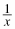+ 20, find the following: Homework Help ✎

1. 3f(x)

2. −2f(x)

3.4.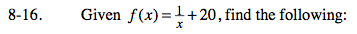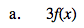Multiply each term by 3.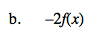Multiply each term by −2.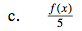Divide each term by 5.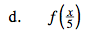$\text{Substitute for each } x \text{ in the function the expression }\frac{x}{5}.$